# Anti-de Sitter space

complete maximal space-like hypersurfaces in an

Let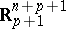be an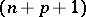-dimensional Minkowski space of index, i.e.,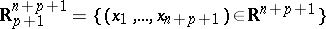and is equipped with the Lorentz metric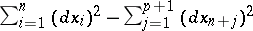. For, let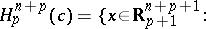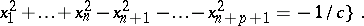Thus,is an-dimensional indefinite Riemannian manifold of indexand of constant curvature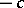. It is called an-dimensional anti-de Sitter space of constant curvatureand of index. A hypersurfaceof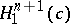is said to be space-like if the metric oninduced by that of ambient spaceis positive definite. The mean curvature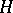ofis defined as in the case of Riemannian manifolds. By definition,is a maximal hypersurface if the mean curvatureofis identically zero. S. Ishihara proved that a complete maximal space-like hypersurfaceinsatisfies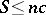, andif and only ifis isometric to the hyperbolic cylinder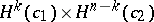, whereis the squared norm of the second fundamental form ofand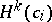,, is a-dimensional hyperbolic space of constant curvature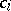. The rigidity of the hyperbolic cylinderinwas proved by U.-H. Ki, H.S. Kim and H. Nakagawa [a3]: for a given integerand constant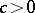, there exists a constant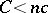, depending onand, such that the hyperbolic cylinderis the only complete maximal space-like hypersurface inof constant scalar curvature and such that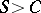. In particular, for, Q.M. Cheng [a1] has characterized the complete maximal space-like hypersurfaces in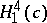under the condition of constant Gauss–Kronecker curvature (cf. Gaussian curvature): Letbe a-dimensional complete maximal space-like hypersurface of. Now:

1) if the Gauss–Kronecker curvature ofis a non-zero constant, thenis the hyperbolic cylinder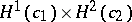;

2) if the scalar curvatureis constant and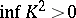, thenis the hyperbolic cylinder. There are no complete maximal space-like hypersurfaces inwith constant scalar curvature and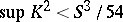.

On the other hand, complete space-like submanifolds in anti-de Sitter spaces with parallel mean curvature have been investigated by many authors.

Cf. also De Sitter space.